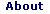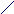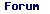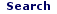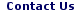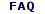Mon - 01 Jun 2020

WELCOME TO RFCABLES.ORG

# Skin Depth Calculator

The well-known equation is for skin depth given below.
Note that skin depth (δ) is a function of only three variables, frequency (ƒ), resistivity (ρ), and relative permeability (μR).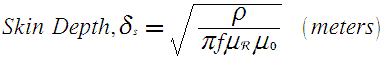Where;
δ = the skin depth in metres
ρ = the resistivity of the medium in ohm-meter,
ƒ = the frequency of the current in Hz,
μR = the relative permeability of the medium,
μ0 = Permeability constant (Henries/meter) = 4π*10-7H/m

 Frequency (GHz)= Resistivity (micro-ohm-centimeters)= Relative Permeability=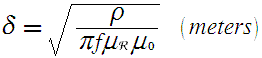Skin Depth (micro meters)= Skin Depth (micro inches)=

The skin depths of some common metals at a frequency of 10 GHz (microwave region) are less than a micron:

Conductor Skin depth (μm)
Aluminum 0.80
Copper 0.65
Gold 0.79
Silver 0.64